# Grover Fixed Point Search for QUBO

### Mentor: Alex Khan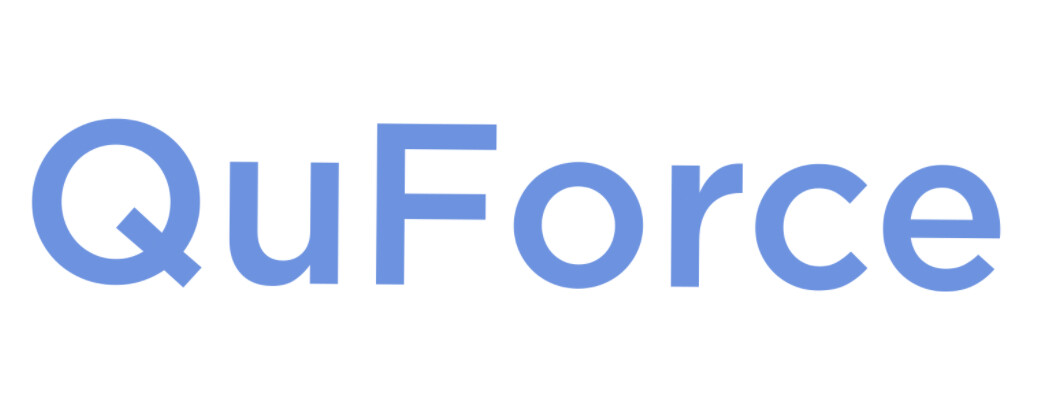# Grover's Search Algorithm

• Built to find a marked state in database of unsorted unmarked ones.

• Done through iterative application of reflections.

• Quadratic speed up as $N$ increases.

### The Soufflé Problem

• Grover's struggles when the number of target states is unknown (the number of iterations is also unknown).

• With too few rotations, we "undercook" the state.

• Too many rotations and we "overcook" the state.

### Solution: Grover Fixed Point Search

• A generalization of Grover's Search Algorithm.

• Yoder–Low–Chuang (2014): Phases are added to the reflection oracles based on success probability.

• As the number of iterations increase, the probability of success can only increase.

• Eliminates the soufflé problem and maintains the quadratic speed up.

# Quadratic Unconstrained Binary Optimization (QUBO) problems

• Problem: given a quadratic (real-valued) function, $f$, on $n$ (classical) bits, find its maximum.

• Such a function is given by a symmetric $n$-by-$n$ matrix, $Q$, and a constant $c$. (WLOG: set $c = 0$.)

• MaxCut is QUBO with $Q =$ Graph Laplacian.

### Circuit design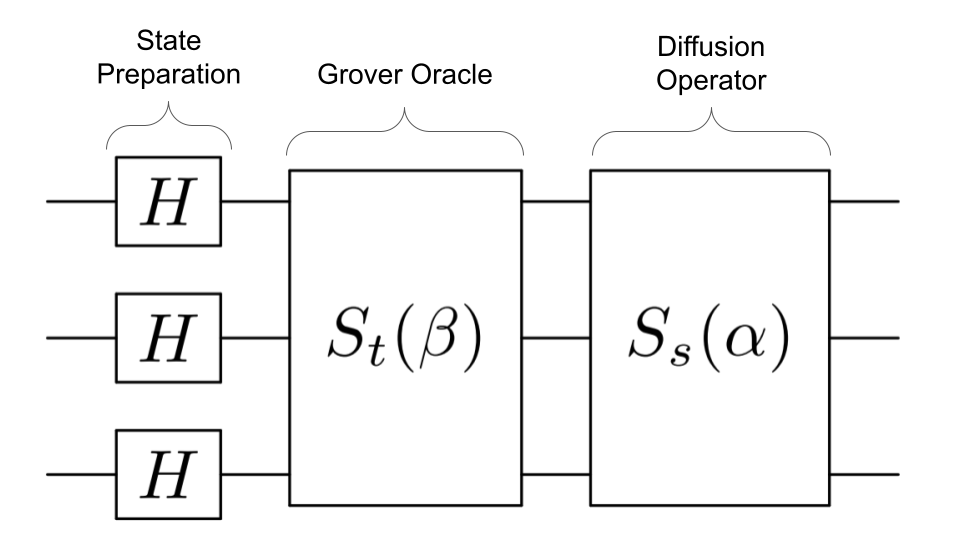• State Preparation: Equal superposition of solutions and ancillas

• Grover Oracle: Marks desired states with some phase adjusted based on the success probability

• Diffusion Operator: Amplifies marked state

• The last two steps are repeated for all angles $\alpha$ and $\beta$.

### Oracle Overview

• Digitize values into ancillas.

• Mark desired states (first ancilla becomes 1 when value is above or on threshold).

• Reset ancillas to threshold value.

• Based on Gilliam–Woerner–Gonciulea (2021): $S_t$ uses $O \left( \ln \left( m \right) \right)$ ancillas.

### Example: MaxCut

• For a graph, $G = (V, E)$, find $S \subset V$ so that \begin{equation} \mathrm{cut}_G (S) = \left| \left\{ (v, v^\prime) \in E : v \in S \: \& \: v^\prime \notin S \right\} \right|, \end{equation} is maximal.

•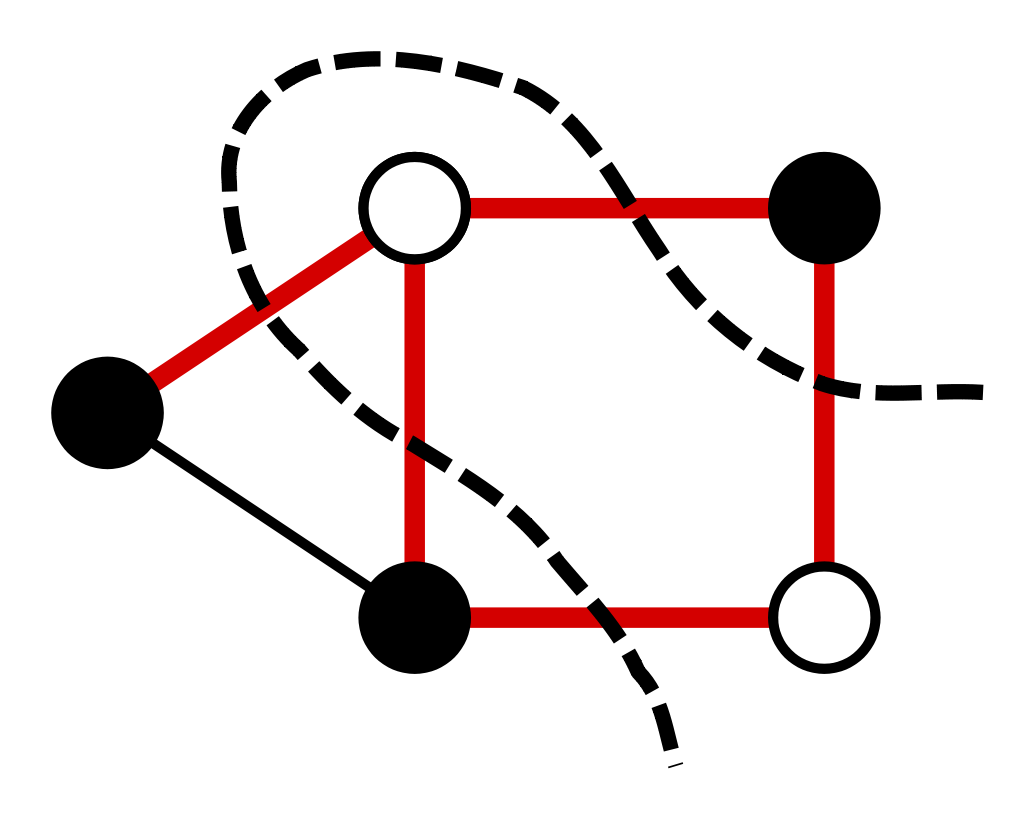• Recall that MaxCut is QUBO with $Q =$ Graph Laplacian.

• MaxCut is NP-hard.

• We only have polynomial time MaxCut-approximations.

• Goemans–Williamson Algorithm: polynomial time algorithm to get a cut at least $\approx 88$% of MaxCut.

• Erdős–Edwards bound for the maximal cut of connected graphs: \begin{equation} \mathrm{MaxCut}_G \geqslant \tfrac{2 m + n - 1}{4}, \quad \left( n = |V|, \: m = |E| \right). \end{equation} Can be found in $O \left( n^4 \right)$ time.

### Results

• For graphs less than or equal to 20 verticies and with target probability $\geqslant 90$%, $l = 1, 2$ suffices.

• Simulation with the noise model of a modern, all-to-all connected Quantum Computer (IonQ's Harmony) suggests that the noise of either oracles destroys most of the results when $n \geqslant 6$.

• This all works for any QUBO problem with minimal modification.

• In fact, works for all binary optimization problems (short term #1).

• Note: we pre-computed the exact ratios of marked states; in lieu of that, one can also use an adaptive version (short term #2).

# Example

## Erdős–Rényi-graph with $p = 0.5$, $|V| = 20$, & $|E| = 101$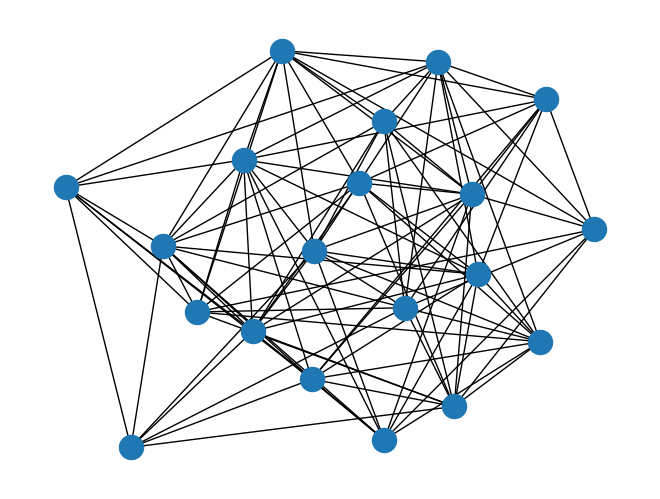# Simulation without noise

 vertices 20 edges 101 $\mathrm{MaxCut}$ 66 Erdős–Edwards 56 random chance 13.98% $\delta$ 0.93 Query complexity 1 success probability 80.89% expectation 55.87 (+5.37) ancillas 7 gate count 8097 circuit depth 4009 simulation time $\sim$ 2 minutes

# cut distributions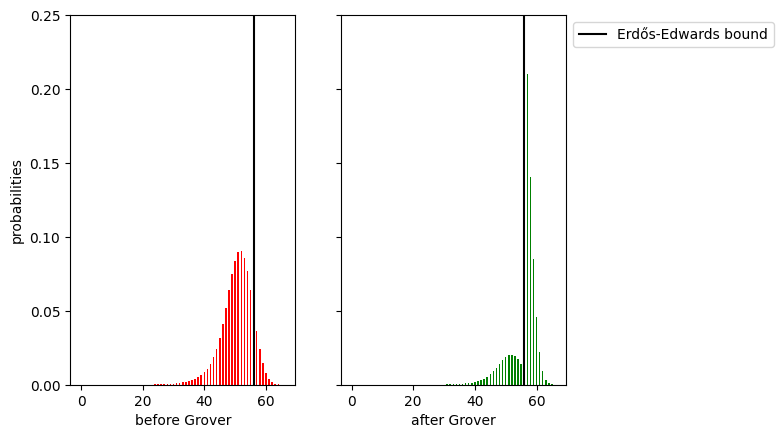### Future plans/ideas

• Benchmark against existing algorithms.

• Refine and study the adaptive variant.

• Math problem: given a QUBO instance, can we bound the ratio of good configurations polynomially, from below?

• More sci-fi: This construction can be used as an (approximate) Grover mixer for QAOA.

• The oracle design can be used immediately for Threshold-QAOA (cf. Golden–Bärtschi–O’Malley–Eidenbenz, 2021).